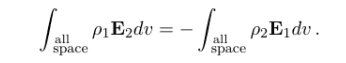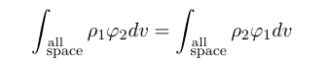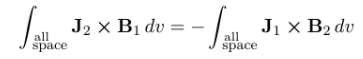# Green's reciprocity theorem about current density and magnetic field

• SanaiBongchul

#### SanaiBongchul

Homework Statement
I have to prove the Green's reciprocity theorem about the Electric field, electric potential, and magnetic field.
Relevant Equations
Intgral over all space J_2 cross B_1 = -Integral over all space J_1 cross B_2I have to prove three equations above.
For first two equations, I've been thought and made reasonable answer by using a definition of the electricfield.
However, for third, I can't use a definition of a magnetic field due to the cross product
Like J_2 X J_1 X (r_2 - r_1).
I think three of 'em are kinda related to Newton's third law of lorentz force.
Does anyone know about the proof?
Or would you think you can prove these?

•PhDeezNutz
A:The third equation is simply a vector identity, and can be proved by expanding the cross products. $$\mathbf{J}_2 \times \mathbf{J}_1 \times (\mathbf{r}_2 - \mathbf{r}_1) = (\mathbf{r}_2 - \mathbf{r}_1)\cdot(\mathbf{J}_2 \times \mathbf{J}_1) - (\mathbf{J}_2 \cdot (\mathbf{r}_2 - \mathbf{r}_1))\mathbf{J}_1 + (\mathbf{J}_1 \cdot (\mathbf{r}_2 - \mathbf{r}_1)) \mathbf{J}_2$$The left-hand side of the equation is clearly zero, so the two terms on the right-hand side must also be equal to zero. The first term is equal to zero because the dot product of two vectors perpendicular to each other is zero. The second and third terms are equal to zero because $\mathbf{J}_2$ and $\mathbf{J}_1$ are constant, so they cannot depend on $\mathbf{r}_2 - \mathbf{r}_1$.